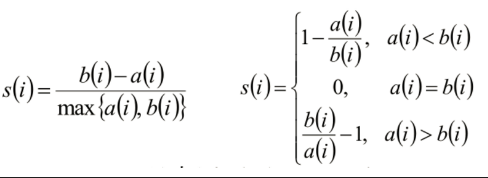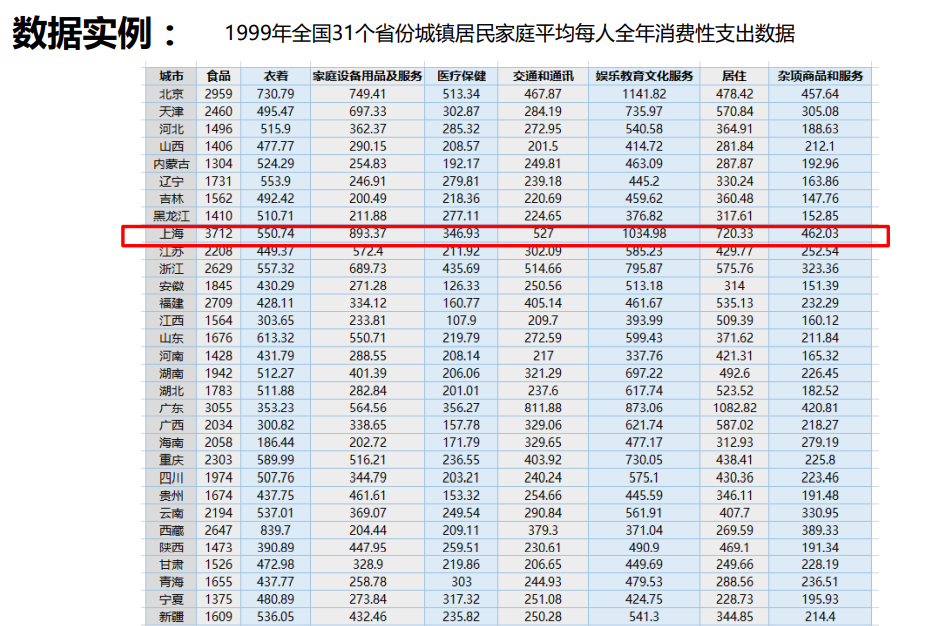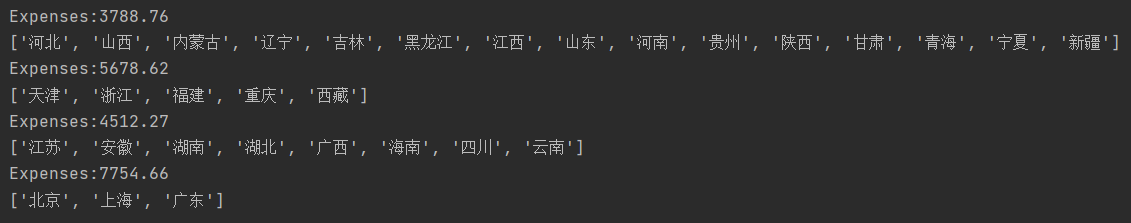• 学习笔记(09):第四章：非监督学习-聚类案例分析：Event聚类
2020-02-26 19:26:43

normalize是以行为单位，进行标准画非监督学习 机器学习 人工智能
更多相关内容
• km2=KMeans(n_clusters=2).fit(X) #查看km聚类完每个数据属于那个类别 print(km.labels_) beer['cluster']=km.labels_ beer['cluster2']=km2.labels_ beer=beer.sort_values('cluster') # print(beer) from pandas....
import pandas as pd
# print(bear)
#取出四列数据
X=beer[['calories','sodium','alcohol','cost']]

#导入kmeans
from sklearn.cluster import KMeans

km=KMeans(n_clusters=3).fit(X)
km2=KMeans(n_clusters=2).fit(X)
#查看km聚类完每个数据属于那个类别
print(km.labels_)

beer['cluster']=km.labels_
beer['cluster2']=km2.labels_
beer=beer.sort_values('cluster')
# print(beer)

from pandas.plotting import scatter_matrix
cluster_centers=km.cluster_centers_
cluster_centers_2=km2.cluster_centers_

beer_mean=beer.groupby('cluster').mean()
print(beer_mean)

#找出中心点
centers=beer.groupby('cluster').mean().reset_index()
import matplotlib.pyplot as plt
plt.rcParams['font.size']=14

import numpy as np

colors=np.array(['red','green','blue','yellow'])

plt.scatter(beer["calories"], beer["alcohol"],c=colors[beer["cluster"]])

plt.scatter(centers.calories, centers.alcohol, linewidths=3, marker='+', s=300, c='black')

plt.xlabel("Calories")
plt.ylabel("Alcohol")
plt.show()
#3个簇的结果
scatter_matrix(beer[["calories","sodium","alcohol","cost"]],s=100, alpha=1, c=colors[beer["cluster"]], figsize=(10,10))
plt.suptitle("With 3 centroids initialized")
plt.show()

#前面没有对数据做标准化的处理
from sklearn.preprocessing import StandardScaler
scaler=StandardScaler()
X_scaled=scaler.fit_transform(X)
# print(X_scaled)
km1=KMeans(n_clusters=3).fit(X_scaled)
beer['scaled_cluster']=km1.labels_
beer=beer.sort_values('scaled_cluster')
print(beer)

#聚类评估轮廓系数
from sklearn import metrics
score_scaled = metrics.silhouette_score(X,beer.scaled_cluster)
score = metrics.silhouette_score(X,beer.cluster)
print(score_scaled, score)

#簇系数
scores = []
for k in range(2,20):
labels = KMeans(n_clusters=k).fit(X).labels_
score = metrics.silhouette_score(X, labels)
scores.append(score)

print(scores)

plt.plot(list(range(2,20)), scores)
plt.xlabel("Number of Clusters Initialized")
plt.ylabel("Sihouette Score")
plt.show()

#DBSCAN  算法
from sklearn.cluster import DBSCAN
db=DBSCAN(eps=10,min_samples=2).fit(X)
labels = db.labels_
beer['cluster_db'] = labels
beer=beer.sort_values('cluster_db')
print(beer)
#重复上面步骤可以看到标准化过后的数据情况



## 聚类评估：轮廓系数（Silhouette Coefficient ）计算样本i到同簇其他样本的平均距离ai。ai 越小，说明样本i越应该被聚类到该簇。将ai 称为样本i的簇内不相似度。
计算样本i到其他某簇Cj 的所有样本的平均距离bij，称为样本i与簇Cj 的不相似度。定义为样本i的簇间不相似度：bi =min{bi1, bi2, …, bik}
si接近1，则说明样本i聚类合理
si接近-1，则说明样本i更应该分类到另外的簇
若si 近似为0，则说明样本i在两个簇的边界上。

展开全文• python 文本聚类分析案例说明摘要1、结巴分词2、去除停用词3、生成tfidf矩阵4、K-means聚类5、获取主题词 / 主题词团 说明 实验要求：对若干条文本进行聚类分析，最终得到几个主题词团。 实验思路：将数据进行预处理...
•文档
• kmeans聚类案例，包含测试的模拟数据xml文件，kmeans聚类分析代码
• 1 sklearn层次聚类 01 ward  最小化所有聚类内的平方差总和，这是一种方差最小化的优化方向，这是与k-means的目标韩式相似的优化方法，但是用聚类分层的方法处理。 02 Maximum 或者complete linkage...2 案例 ...

1 sklearn层次聚类

01 ward

最小化所有聚类内的平方差总和，这是一种方差最小化的优化方向，这是与k-means的目标韩式相似的优化方法，但是用聚类分层的方法处理。

2 案例

import pandas as pd

index_col='客户编号')

# 提取关键特征 data.columns

fColumns=[ '工作日上班时电话时长', '工作日下半时电话时长', '周末电话时长', '国际电话时长']

# 数据标准化0-1标准化

data=data[fColumns]

data[fColumns]=round(
(data[fColumns]-data[fColumns].min())/(data[fColumns].max()-data[fColumns].min()),
3)

# 导入层次聚类函数

P=dendrogram(Z,0) # 画谱系聚类图

''' 只展示12个数据
P1=dendrogram(Z,
truncate_mode='lastp',
p=12,
leaf_font_size=12.
)

'''

# 导入sklearn的层次聚类函数

from sklearn.cluster import AgglomerativeClustering

k=3

model.fit(data[fColumns]) # 训练模型

# 详细输出原始数据极其类别

r=pd.concat([data,pd.Series(model.labels_,index=data.index)],axis=1)

r.columns=list(data.columns)+['聚类类别']

import matplotlib.pyplot as plt

plt.rcParams['font.sans-serif']=['SimHei'] #用来正常显示中文标签
plt.rcParams['axes.unicode_minus']=False #用来正常显示负号
#有中文出现的情况，需要u'内容'

style=['ro-','go-','bo-']

xlabels=fColumns

# # %matplotlib qt 弹窗

for i in range(k): #注意作图、做出不同样式

plt.figure()

tmp=r[r[u'聚类类别']==i].iloc[:,:4] # 提取每一类

for j in range(len(tmp)):

plt.plot(range(1,5),tmp.iloc[j],style[i])

plt.xticks(range(1,5),xlabels,rotation=20) #坐标标签

plt.savefig(u'%s%s,png'%('D:\\DATA\\pycase\\7.1\\data',i)) #保存图片

展开全文• Kmeans聚类方法原理： 1.首先随机定出K个聚类中心； 2.计算数据中每一个点到K个聚类中心的距离（欧氏距离），哪个最小就把这个点归到哪一个簇中； 3.计算每一个簇中所有点的中心点（向量对应元素取平均），这些点...

Kmeans聚类方法原理：
1.首先随机定出K个聚类中心；
2.计算数据中每一个点到K个聚类中心的距离（欧氏距离），哪个最小就把这个点归到哪一个簇中；
3.计算每一个簇中所有点的中心点（向量对应元素取平均），这些点确定为新的聚类中心；
4.重复步骤2、3，直到所有的聚类中心不再发生变化。

知道这个原理后，我们应用此来做一个简单的小例子。
需要安装的第三方库：
numpy、matplotlib、scipy、sklearn（注意顺序不要错哦，后三个库是以numpy为基础，sklearn右是以前三个为基础的）
安装方式（pip安装）：
对于不会翻墙的小伙伴，可以从镜像源网站下载，速度同样非常快，打开DOS窗口，以numpy为例，复制以下代码即可：

pip install numpy -i http://pypi.douban.com/simple/ --trusted-host pypi.douban.com


由于dos窗口里没法把光标移到中间删改东西，所以大家可以把这一行代码保存到一个word文档里，每次需要安装一个库时，就先把这行里面的名字改掉再复制到命令行窗口就可以啦。

数据介绍：
现有1999年全国31个省份城镇居民家庭平均每人全年消费性支出的八个主
要变量数据，这八个变量分别是：食品、衣着、家庭设备用品及服务、医疗
保健、交通和通讯、娱乐教育文化服务、居住以及杂项商品和服务。利用已
有数据，对31个省份进行聚类。
实验目的：
通过聚类，了解1999年各个省份的消费水平在国内的情况。
数据展示：数据链接：
链接：https://pan.baidu.com/s/1PArczd8hxp1AbQe9MOKwgQ
提取码：9fg2
（将这个txt文档跟下面的程序放到一个文件夹里哦）
程序展示：
为了便于理解，在一些地方我添加了注释进行说明。

import numpy as np
from sklearn.cluster import KMeans

#该函数为从txt中读取数据
fr = open(filePath, 'r+')
retData = []
retCityName = []
for line in lines:
items = line.strip().split(",")#strip()函数默认参数删除空白符（包括'\n', '\r', '\t',  ' ')
#line本来是字符串，被.split(",")后，成了若干个字符串组成的列表了
retCityName.append(items)
retData.append([float(items[i]) for i in range(1, len(items))])#注意此处添加的是一个列表
return retData, retCityName

if __name__ == '__main__':
# 一个python文件通常有两种使用方法，第一是作为脚本直接执行，第二是 import 到其他的 python 脚本中被调用（模块重用）执行。
# 因此 if __name__ == 'main': 的作用就是控制这两种情况执行代码的过程，在 if __name__ == 'main': 下的代码只有在
# 第一种情况下（即文件作为脚本直接执行）才会被执行，而 import 到其他脚本中是不会被执行的。
km = KMeans(n_clusters=4)
label = km.fit_predict(data)#fit_predict()：计算簇中心以及为簇分配序号，label：聚类后各数据所属的标签
expenses = np.sum(km.cluster_centers_, axis=1)
# 当axis为0时,是压缩行,即将每一列的元素相加,将矩阵压缩为一行，当axis为1时,是压缩列,即将每一行的元素相加,将矩阵压缩为一列
# 此处即是将聚类中心的各项数据求和
# print(expenses)
CityCluster = [[], [], [], []]
for i in range(len(cityName)):
CityCluster[label[i]].append(cityName[i])
#label中的每个元素都与cityname对应，也就是把每个城市名都添加到对应的簇中
for i in range(len(CityCluster)):
print("Expenses:%.2f" % expenses[i])
print(CityCluster[i])


运行结果：资料来源：
mooc北京理工大学python机器学习

展开全文kmeans sklearn python 机器学习
•数据挖掘
• 基于AGNES聚类的能耗均衡WSNs优化路由算法.pdf
• 提出了一种基于DTW的符号化时间序列聚类算法，对降维后得到的不等长符号时间序列进行聚类。该算法首先对时间序列进行降维处理，提取时间序列的关键点，并对其进行符号化；其次利用DTW方法进行相似度计算；最后利用...
• KMeans类：K-means 算法的实现类 Vectors：向量 创建案例类 case class Iris(features: org.apache.spark.ml.linalg.Vector) Vector 是一个容器，可以保存其他数据对象，也称为集合。 Vector 是Scala标准包的一部分...spark
• 层次聚类 -- coding: utf-8 -- “”" @author: CDA教研组 申明：CDA版权所有 官网：edu.cda.cn “”" 第一步：手动测试主成分数量 1、导入数据 （CDA版权所有） import pandas as pd model_data = pd.read_csv(...数据挖掘 机器学习
• 这篇文章从对聚类的感性认识到聚类算法的实现：k个初始中心点的选择，中心点的迭代，直到算法收敛得到聚类结果。但有几个问题需要回答：如何判断数据是否适合聚类？k类是如何确定的？遇到数据集小的...
• isodata的matlab代码博客 ISODATA-clustering-python isodata clustering algorithm implemented in python
• 1.首先我们需要选择一个k值，也就是我们希望把数据分成多少类，这里k值的选择对结果的影响很大，Ng的课说的选择方法有两种一种是elbow method，简单的说就是根据聚类的结果和k的函数关系判断k为多少的时候效果最好。...
• 目录1、分层聚类算法2、方法3、分析步骤4、案例1、分层聚类算法层次聚类算法又称为树聚类算法，它根据数据之间的距离，透过一种层次架构方式，反复将数据进行聚合，创建一个层次以分解给定的数据集。2、方法01 聚类...
• 聚类 今天说K-means聚类算法，但是必须要先理解聚类和分类的区别，很多业务人员在日常分析时候不是很严谨，混为一谈，其实二者有本质的区别。 分类其实是从特定的数据中挖掘模式，作出判断的过程。比如Gmail邮箱里有...
• DBSCAN 是一种非常著名的基于密度的聚类算法。其英文全称是 Density-Based Spatial Clustering of Applications with Noise，意即：一种基于密度，对噪声鲁棒的空间聚类算法。直观效果上看，DBSCAN 算法可以找到样本...
• 作者：Pulkit Sharma 翻译：陈超 校对：吴振东本文约4700字，建议阅读15分钟本文从对比无监督学习和监督学习的特征切入，结合具体的案例来给大家介绍层级聚类的概念、应用场景、主要类型以及Python实现。引言理解...
• Python DBSCAN聚类算法 使用DBSCAN算法的图像像素聚类Python
• 面试前，跑了一下kmens算法，里面有一个人总结的文档，希望对大家有帮助
• center = km.cluster_centers_ # 聚类中心值# show_res_km(data.values,y_predict,center) print(center) radarmap(center,k) radar_plt() 雷达图如下：  对数据分析结果进行散点图绘制的方法有......